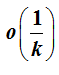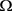# 一种非光滑强凸函数的随机次梯度镜面下降算法A Stochastic Sub-Gradient Mirror Descent Algorithm for Non-Smooth and Strongly Convex Functions

• 全文下载: PDF(477KB)    PP.221-229   DOI: 10.12677/PM.2018.83028
• 下载量: 520  浏览量: 1,884   国家自然科学基金支持

Mirror descent (MD) has been widely used to deal with the machine learning problems. For large scale data processing and non-smooth loss convex optimization problem, we proposed an improved mirror descent method, which is called modified stochastic sub-gradient mirror descent method. It combined an iterative average method with stochastic sub-gradient descent method. In the process of weighted average, the average iteration is not used to construct the algorithm, but occurs as a byproduct of our algorithm. The average weight is determined by the step size used by the algorithm. Our algorithm has good convergence. For strong convex functions, we show that the optimal convergence rate of the algorithm arrives at.

1. 引言

$\underset{w\in \Omega }{\mathrm{min}}F\left(w\right)=E\left[f\left(w,\xi \right)\right]$ . (1)

$F\left(w\right)+〈g\left(w\right),u-w〉\le F\left(u\right)$ ，对任意的 $u\in \Omega$

2. 基本的镜面下降算法

$B\left(·,·\right)$ 表示集合 $\Omega$ 中任意两点的Bregman距离函数，一个基本的镜面下降算法使用了一个非线性投影序列：

${w}_{k+1}=\underset{w\in \Omega }{\mathrm{arg}\mathrm{min}}\left\{〈{g}_{k},w〉+\frac{1}{{\alpha }_{k}}{B}_{\phi }\left(w,{w}_{k}\right)\right\}$ .

MD方法要求距离函数的范数满足如下性质   ：

1) 空间E上的范数 $‖·‖$ 嵌入于 $\Omega$ ，且 ${E}^{*}$ 上的对偶范数 ${‖•‖}_{*}$ 满足：

${‖\xi ‖}_{*}=\underset{w}{\mathrm{max}}\left\{〈\xi ,w〉:‖w‖\le 1\right\}$ ;

2) 集合上的距离生成函数及范数 $‖·‖$ ，即有连续凸函数 $\phi \left(w\right):\Omega \to R$ 使得：

- 存在 $\phi \left(·\right)$ 的一个次梯度 ${\phi }^{\prime }\left(·\right)$ ，在集合 ${\Omega }^{0}=\left\{w\in \Omega :\partial \phi \left(w\right)\ne \varnothing \right\}$ 上连续；

- $\phi \left(·\right)$ 关于1范数 $‖·‖$ 是强凸的：

$\forall \left(w,{w}^{\prime }\in {\Omega }^{0}\right):〈\phi \left(w\right)-\phi \left({w}^{\prime }\right),w-{w}^{\prime }〉\ge {‖w-u‖}^{2}$ .

${F}^{*}-F\left(\stackrel{¯}{w}\right)\le \frac{L\sqrt{2V}}{\sqrt{k}}$ .

3. 随机镜面下降算法

$F\left(u\right)\ge F\left(w\right)+〈g\left(w\right),u-w〉+\frac{{\mu }_{F}}{2}{‖u-w‖}^{2}$ , 对任意 $u,w\in \Omega$

$\phi \left(\cdot \right)$ 为集合 $\Omega$ 上连续可微的强凸函数，即存在 ${\mu }_{\phi }>0$ ，使得

$\phi \left(v\right)\ge \phi \left(w\right)+〈\nabla \phi \left(w\right),v-w〉+\frac{{\mu }_{\phi }}{2}{‖v-w‖}^{2}$ , 对任意 $w,v\in \Omega$ (2)

${B}_{\phi }\left(w,u\right)=\phi \left(u\right)-\phi \left(w\right)-〈\nabla \phi \left(w\right),u-w〉$ , 对任意 $u,w\in \Omega$ (3)

${B}_{\phi }\left(w,u\right)-{B}_{\phi }\left(v,u\right)={B}_{\phi }\left(w,v\right)+〈\nabla \phi \left(v\right)-\nabla \phi \left(w\right),u-w〉$ , 对任意 $u,v,w\in \Omega$ (4)

${B}_{\phi }\left(w,u\right)\ge \frac{{\mu }_{\phi }}{2}{‖w-u‖}^{2}$ , 对任意 $u,w\in \Omega$ (5)

${\nabla }_{w}{B}_{\phi }\left(w,u\right)=\nabla \phi \left(u\right)-\nabla \phi \left(w\right)$ 。对任意 $u,w\in \Omega$ (6)

${w}_{0}\in \Omega$ 为初始点，则问题(1)的次梯度镜面下降法的迭代公式如下：

${w}_{k+1}=\underset{w\in \Omega }{\mathrm{arg}\mathrm{min}}\left\{{\alpha }_{k}〈{g}_{k},w-{w}_{k}〉+{B}_{\phi }\left({w}_{k},w\right)\right\}$ , 对任意 $k>0$

${w}_{k+1}=\underset{w\in \Omega }{\mathrm{arg}\mathrm{min}}\left\{{\alpha }_{k}〈{\stackrel{˜}{g}}_{k},w-{w}_{k}〉+{B}_{\phi }\left({w}_{k},w\right)\right\}$ , 对任意 $k>0$ (7)

$\frac{1-{\alpha }_{k+1}}{{\alpha }_{k+1}^{2}}\le \frac{1}{{\alpha }_{k}^{2}}$ , 对任意 $k\ge 0$${\alpha }_{0}=1$ . (8)

$0<{\alpha }_{k+1}\le \frac{\sqrt{{\alpha }_{k}^{4}+4{\alpha }_{k}^{2}}-{\alpha }_{k}^{2}}{2}$ , 对任意 $k\ge 0$

${\alpha }_{k}=\frac{1}{k+1}$${\alpha }_{k+1}=\frac{\sqrt{{\alpha }_{k}^{4}+4{\alpha }_{k}^{2}}-{\alpha }_{k}^{2}}{2}$ , 对任意 $k\ge 0$ (9)

${t}_{k+1}=\frac{\sqrt{1+4{t}_{k}^{2}}+1}{2}$ , 对任意 $k\ge 0$

${t}_{0}=1$  。通过归纳，我们可以发现 ${t}_{k+1}=\frac{k+2}{2}$ ，对任意 $k\ge 0$ ，从而推出(9)中的两个步长公式都满足：

$0<{\alpha }_{k}\le \frac{2}{k+2}$ , 对任意 $k\ge 0$ , 且 ${\alpha }_{0}=1$

${\alpha }_{k}^{2}\ge 1/\left({\sum }_{t=0}^{k}\frac{1}{{\alpha }_{t}}\right)$ , 对任意 $k\ge 0$ .

a) 任意选取初始点 ${w}_{0}\in \Omega$ ，满足 $E\left[{‖{w}_{0}‖}^{2}\right]\in \Omega <\infty$ ，一般我们选取 ${w}_{0}=\mathrm{arg}{\mathrm{min}}_{w\in \Omega }\phi \left(w\right)$

b) ${w}_{k+1}=\underset{w\in \Omega }{\mathrm{arg}\mathrm{min}}\left\{{\alpha }_{k}〈{\stackrel{˜}{g}}_{k},w-{w}_{k}〉+{B}_{\phi }\left({w}_{k},w\right)\right\}$

c) ${\stackrel{^}{w}}_{k}={\left({\sum }_{t=0}^{k}\frac{1}{{\alpha }_{t}}\right)}^{-1}{\sum }_{t=0}^{k}\left(\frac{1}{{\alpha }_{t}}{w}_{t}\right)$

${\stackrel{^}{w}}_{k}=\frac{{S}_{k}}{{S}_{k+1}}{\stackrel{^}{w}}_{k-1}+\left(1-\frac{{S}_{k}}{{S}_{k+1}}\right){w}_{k}$ .

4. 算法的收敛性分析

${B}_{\phi }\left(w,u\right)\le \frac{1}{2}{‖w-u‖}^{2}$ , 对任意 $w,u\in \Omega$ (10)

$〈\nabla \phi \left(w\right)-\nabla \phi \left(u\right),w-u〉\le \frac{1}{2}{‖w-u‖}^{2}$ , 对任意 $w,u\in \Omega$

$\frac{1}{2}{‖w-u‖}^{2}\ge 〈\nabla \phi \left(w\right)-\nabla \phi \left(u\right),w-u〉\ge \phi \left(u\right)-\phi \left(w\right)-〈\nabla \phi \left(w\right),w-u〉={B}_{\phi }\left(w,u\right)$ ,

$〈\nabla \stackrel{˜}{\phi }\left(w\right)-\nabla \stackrel{˜}{\phi }\left(u\right),w-u〉\le L{‖w-u‖}^{2}$ , 对任意 $w,u\in \Omega$

$\stackrel{˜}{g}\left(w\right)=g\left(w\right)$ 时，假设1中次梯度在 $\Omega$ 上一致有界，即存在 $C>0$ 使得对任意 $w\in \Omega$ ，有 $‖g\left(w\right)‖\le C$ 。若目标函数的次梯度一致有界，且满足 $E\left[\stackrel{˜}{g}\left(w\right)|w\right]=g\left(w\right)$ ，存在某个 $\gamma >0$ ，使得对任意 $w\in \Omega$$E\left[{‖\stackrel{˜}{g}\left(w\right)-g\left(w\right)‖}_{*}^{2}|w\right]\le {\gamma }^{2}$ ，则对于一般范数，假设1成立，且 ${\stackrel{˜}{C}}^{2}=2{C}^{2}+2{\gamma }^{2}$ 。显然欧式范数下有同样结论。

${\Gamma }_{k}=\sigma \left\{{w}_{0},{\stackrel{˜}{g}}_{0},\cdots ,{\stackrel{˜}{g}}_{k-1}\right\}$ , 对任意 $k\ge 1$ ,

${\Gamma }_{0}=\sigma \left\{{w}_{0}\right\}$ 。根据σ-场，在假设1下，我们得到随机次梯度镜面下降法生成的序列 $\left\{{w}_{k}\right\}$ 有如下基本性质：

$E\left[{B}_{\phi }\left({w}_{k+1},u\right)|{\Gamma }_{k}\right]+{\alpha }_{k}〈{g}_{k},{w}_{k}-u〉\le {B}_{\phi }\left({w}_{k},u\right)+\frac{{\alpha }_{k}^{2}{\stackrel{˜}{C}}^{2}}{2{\mu }_{\phi }}$ .

$0\le 〈{\alpha }_{k}{\stackrel{˜}{g}}_{k}+{\nabla }_{u}{B}_{\phi }\left({w}_{k},{w}_{k+1}\right),u-{w}_{k+1}〉$ ，对任意 $u\in \Omega$

$0\le 〈{\alpha }_{k}{\stackrel{˜}{g}}_{k}+\nabla \phi \left({w}_{k+1}\right)-\nabla \phi \left({w}_{k}\right),u-{w}_{k+1}〉$ , 对任意 $u\in \Omega$

${\alpha }_{k}〈{\stackrel{˜}{g}}_{k},{w}_{k+1}-u〉\le 〈\nabla \phi \left({w}_{k+1}\right)-\nabla \phi \left({w}_{k}\right),u-{w}_{k+1}〉$ . (11)

$〈\nabla \phi \left({w}_{k+1}\right)-\nabla \phi \left({w}_{k}\right),u-{w}_{k+1}〉={B}_{\phi }\left({w}_{k},u\right)-{B}_{\phi }\left({w}_{k+1},u\right)-{B}_{\phi }\left({w}_{k},{w}_{k+1}\right)$ .

${\alpha }_{k}〈{\stackrel{˜}{g}}_{k},{w}_{k+1}-u〉\le {B}_{\phi }\left({w}_{k},u\right)-{B}_{\phi }\left({w}_{k+1},u\right)-{B}_{\phi }\left({w}_{k},{w}_{k+1}\right)$ .

$\phi \left(w\right)$ 的强凸性质，以及式(5)可推出，

${\alpha }_{k}〈{\stackrel{˜}{g}}_{k},{w}_{k+1}-u〉\le {B}_{\phi }\left({w}_{k},u\right)-{B}_{\phi }\left({w}_{k+1},u\right)-\frac{{\mu }_{\phi }}{2}{‖{w}_{k}-{w}_{k+1}‖}^{2}$ . (12)

$\begin{array}{l}{\alpha }_{k}〈{\stackrel{˜}{g}}_{k},{w}_{k+1}-u〉={\alpha }_{k}〈{\stackrel{˜}{g}}_{k},{w}_{k+1}-{w}_{k}〉+{\alpha }_{k}〈{\stackrel{˜}{g}}_{k},{w}_{k}-u〉\\ \ge -|〈\frac{{\alpha }_{k}}{\sqrt{{\mu }_{\phi }}}{\stackrel{˜}{g}}_{k},\sqrt{{\mu }_{\phi }}\left({w}_{k+1}-{w}_{k}\right)〉|+{\alpha }_{k}〈{\stackrel{˜}{g}}_{k},{w}_{k}-u〉\\ \ge -\frac{{\alpha }_{k}^{2}}{2{\mu }_{\phi }}{‖{\stackrel{˜}{g}}_{k}‖}_{*}^{2}-\frac{{\mu }_{\phi }}{2}{‖{w}_{k+1}-{w}_{k}‖}^{2}+{\alpha }_{k}〈{\stackrel{˜}{g}}_{k},{w}_{k}-u〉\end{array}$ , (13)

${‖•‖}_{*}$ 为范数 $‖•‖$ 的共轭范数。消去 ${‖{w}_{k+1}-{w}_{k}‖}^{2}$ ，我们有

${\alpha }_{k}〈{\stackrel{˜}{g}}_{k},{w}_{k}-u〉\le {B}_{\phi }\left({w}_{k},u\right)-{B}_{\phi }\left({w}_{k+1},u\right)+\frac{{\alpha }_{k}^{2}}{2{\mu }_{\phi }}{‖{\stackrel{˜}{g}}_{k}‖}_{*}^{2}$ .

${\Gamma }_{k}$ 上取条件期望，再根据假设1，我们得到

${\alpha }_{k}〈{\stackrel{˜}{g}}_{k},{w}_{k}-u〉\le {B}_{\phi }\left({w}_{k},u\right)-E\left[{B}_{\phi }\left({w}_{k+1},u\right)|{\Gamma }_{k}\right]+\frac{{\alpha }_{k}^{2}{\stackrel{˜}{C}}^{2}}{2{\mu }_{\phi }}$ .

$E\left[{B}_{\phi }\left({w}_{k+1},{w}_{*}\right)\right]+\frac{1}{{\mu }_{F}}\frac{1}{\left({\sum }_{t=0}^{k}\frac{1}{{\alpha }_{t}}\right)}{\sum }_{t=0}^{k}\frac{1}{{\alpha }_{t}}\left(E\left[F\left({w}_{t}\right)\right]-F\left({w}_{*}\right)\right)\le \left(k+1\right)\frac{{\alpha }_{k}^{2}{\stackrel{˜}{C}}^{2}}{2{\mu }_{F}^{2}{\mu }_{\phi }}$ .

$E\left[{B}_{\phi }\left({w}_{k+1},{w}_{*}\right)|{\Gamma }_{k}\right]+\frac{{\alpha }_{k}}{{\mu }_{F}}〈{g}_{k},{w}_{k}-{w}_{*}〉\le {B}_{\phi }\left({w}_{k},{w}_{*}\right)+\frac{{\alpha }_{k}^{2}{\stackrel{˜}{C}}^{2}}{2{\mu }_{F}^{2}{\mu }_{\phi }}$ ,

$\frac{{\alpha }_{k}}{{\mu }_{F}}〈{g}_{k},{w}_{k}-{w}_{*}〉\ge \frac{{\alpha }_{k}}{{\mu }_{F}}\left(F\left({w}_{k}\right)-F\left({w}_{*}\right)\right)+\frac{{\alpha }_{k}}{2}{‖{w}_{k}-{w}_{*}‖}^{2}\ge \frac{{\alpha }_{k}}{{\mu }_{F}}\left(F\left({w}_{k}\right)-F\left({w}_{*}\right)\right)+{\alpha }_{k}B\left({w}_{k},{w}_{*}\right)$ .

$E\left[{B}_{\phi }\left({w}_{k+1},{w}_{*}\right)\right]+\frac{{\alpha }_{k}}{{\mu }_{F}}\left(E\left[F\left({w}_{k}\right)\right]-F\left({w}_{*}\right)\right)\le \left(1-{\alpha }_{k}\right)E\left[{B}_{\phi }\left({w}_{k},{w}_{*}\right)\right]+\frac{{\alpha }_{k}^{2}{\stackrel{˜}{C}}^{2}}{2{\mu }_{F}^{2}{\mu }_{\phi }}$ ,(14)

$\begin{array}{l}\frac{1}{{\alpha }_{k}^{2}}E\left[{B}_{\phi }\left({w}_{k+1},{w}_{*}\right)\right]+\frac{1}{{\alpha }_{k}{\mu }_{F}}\left(E\left[F\left({w}_{k}\right)\right]-F\left({w}_{*}\right)\right)\\ \le \frac{\left(1-{\alpha }_{k}\right)}{{\alpha }_{k}^{2}}E\left[{B}_{\phi }\left({w}_{k},{w}_{*}\right)\right]+\frac{{\stackrel{˜}{C}}^{2}}{2{\mu }_{F}^{2}{\mu }_{\phi }}\\ \le \frac{1}{{\alpha }_{k-1}^{2}}E\left[{B}_{\phi }\left({w}_{k},{w}_{*}\right)\right]+\frac{{\stackrel{˜}{C}}^{2}}{2{\mu }_{F}^{2}{\mu }_{\phi }}\end{array}$ .

$\frac{1}{{\alpha }_{k}^{2}}E\left[{B}_{\phi }\left({w}_{k+1},{w}_{*}\right)\right]+\frac{1}{{\mu }_{F}}\underset{t=1}{\overset{k}{\sum }}\frac{1}{{\alpha }_{t}}\left(E\left[F\left({w}_{t}\right)\right]-F\left({w}_{*}\right)\right)\le E\left[{B}_{\phi }\left({w}_{1},{w}_{*}\right)\right]+k\frac{{\stackrel{˜}{C}}^{2}}{2{\mu }_{F}^{2}{\mu }_{\phi }}$ ,(15)

$E\left[{B}_{\phi }\left({w}_{1},{w}_{*}\right)\right]+\frac{1}{{\mu }_{F}}\left(E\left[F\left({w}_{0}\right)\right]-F\left({w}_{*}\right)\right)\le \frac{{\stackrel{˜}{C}}^{2}}{2{\mu }_{F}^{2}{\mu }_{\phi }}$ 。对任意 $k\ge 1$ (16)

$\frac{1}{{\alpha }_{k}^{2}}E\left[{B}_{\phi }\left({w}_{k+1},{w}_{*}\right)\right]+\frac{1}{{\mu }_{F}}{\sum }_{t=0}^{k}\frac{1}{{\alpha }_{t}}\left(E\left[F\left({w}_{t}\right)\right]-F\left({w}_{*}\right)\right)\le \left(k+1\right)\frac{{\stackrel{˜}{C}}^{2}}{2{\mu }_{F}^{2}{\mu }_{\phi }}$ ,

$E\left[{‖{w}_{t}-{w}_{*}‖}^{2}\right]\le \frac{4}{k+1}\frac{{\stackrel{˜}{C}}^{2}}{{\mu }_{F}^{2}{\mu }_{\phi }^{2}}$ , 对任意 $k\ge 0$ ,

$E\left[F\left({\stackrel{^}{w}}_{t}\right)\right]-F\left({w}_{*}\right)\le \frac{2}{k+1}\frac{{\stackrel{˜}{C}}^{2}}{{\mu }_{F}{\mu }_{\phi }}$ , 对任意 $k\ge 0$ ,

$E\left[{‖{\stackrel{^}{w}}_{t}-{w}_{*}‖}^{2}\right]\le \frac{4}{k+1}\frac{{\stackrel{˜}{C}}^{2}}{{\mu }_{F}^{2}{\mu }_{\phi }}$ , 对任意 $k\ge 0$ ,

$E\left[{B}_{\phi }\left({w}_{k+1},{w}_{*}\right)\right]+\frac{1}{{\mu }_{F}}\frac{1}{\left({\sum }_{t=0}^{k}\frac{1}{{\alpha }_{t}}\right)}{\sum }_{t=0}^{k}\frac{1}{{\alpha }_{t}}\left(E\left[F\left({w}_{t}\right)\right]-F\left({w}_{*}\right)\right)\le \left(k+1\right)\frac{{\alpha }_{k}^{2}{\stackrel{˜}{C}}^{2}}{2{\mu }_{F}^{2}{\mu }_{\phi }}$ .

$E\left[{B}_{\phi }\left({w}_{k+1},{w}_{*}\right)\right]+\frac{1}{{\mu }_{F}}\frac{1}{\left({\sum }_{t=0}^{k}\frac{1}{{\alpha }_{t}}\right)}{\sum }_{t=0}^{k}\frac{1}{{\alpha }_{t}}\left(E\left[F\left({w}_{t}\right)\right]-F\left({w}_{*}\right)\right)\le \frac{2}{k+1}\frac{{\alpha }_{k}^{2}{\stackrel{˜}{C}}^{2}}{{\mu }_{F}^{2}{\mu }_{\phi }}$ , (17)

$E\left[F\left({\stackrel{^}{w}}_{k}\right)\right]-F\left({w}_{*}\right)\le \frac{2}{k+1}\frac{{\stackrel{˜}{C}}^{2}}{{\mu }_{F}{\mu }_{\phi }}$ , 对任意 $k\ge 0$ ,

$E\left[{‖{\stackrel{^}{w}}_{k}-{w}_{*}‖}^{2}\right]\le \frac{4}{k+1}\frac{{\stackrel{˜}{C}}^{2}}{{\mu }_{F}^{2}{\mu }_{\phi }}$ , 对任意 $k\ge 0$ ,

$E\left[{B}_{\phi }\left({w}_{k+1},{w}_{*}\right)\right]\le \frac{2}{k+1}\frac{{\stackrel{˜}{C}}^{2}}{{\mu }_{F}^{2}{\mu }_{\phi }}$ , 对任意 $k\ge 0$ ,

$E\left[{‖{w}_{k}-{w}_{*}‖}^{2}\right]\le \frac{4}{k+1}\frac{{\stackrel{˜}{C}}^{2}}{{\mu }_{F}^{2}{\mu }_{\phi }^{2}}$ , 对任意 $k\ge 0$ .

5. 总结

  Juditsky, A. and Nemirovski, A.S. (2010) First Order Methods for Nonsmooth Convex Large-Scale Optimization, II: Utilizing Problem’s Structure. MIT Press, Cambridge.  Ben-Tal, A., Margalit, T. and Nemirovski, A. (2001) The Ordered Subsets Mirror Descent Optimization Method with Applications to Tomography. Society for Industrial and Applied Mathematics, 12, 79-108.  Rakhlin, A., Shamir, O. and Sridharan, K. (2012) Making Gradient Descent Optimal for Strongly Convex Stochastic Optimization. arXiv:1109.5647 [cs.LG]  Beck, A. and Teboulle, M. (2003) Mirror Descent and Nonlinear Projected Subgradient Methods for Convex Optimization. Operations Research Letters, 31, 167-175. https://doi.org/10.1016/S0167-6377(02)00231-6  Duchi, J.C., Shalev-Shwartz, S. and Singer, Y. (2010) Composite Objective Mirror Descent. 23rd Conference on Learning Theory, Haifa, 27-29 June 2010, 14-26.  Sra, S., Nowozin, S. and Wright, S.J. (2011) Optimization for Machine Learning. MIT Press, Cam-bridge.  Brègman, L.M. (1967) The Relaxation Method of Finding the Common Point of Convex Sets and Its Ap-plication to the Solution of Problems in Convex Programming. USSR Computational Mathematics & Mathematical Physics, 7, 200-217. https://doi.org/10.1016/0041-5553(67)90040-7  Tseng, P. (2008) On Accelerated Proximal Gradient Methods for Convex-Concave Optimization. SIAM Journal on Optimization.  陶蔚, 潘志松, 陶卿. 使用Nesterov步长策略投影次梯度方法的个体收敛性[J]. 计算机学报. 2018 (1).  Beck, A. and Te-boulle, M. (2009) A Fast Iterative Shrinkage-Thresholding Algorithm for Linear Inverse Problems. SIAM Journal on Imaging Sciences, 2, 183-202. https://doi.org/10.1137/080716542  Luong, D.V.N., Parpas, P. and Rueckert, D. (2016) A Weighted Mirror Descent Algorithm for Non-Smooth Convex Optimization Problem. Journal of Optimi-zation Theory & Applications, 170, 900-915. https://doi.org/10.1007/s10957-016-0963-5